Electron. J. Diff. Eqns., Vol. 2006(2006), No. 135, pp. 1-8.

### Bifurcation of positive solutions for a semilinear equation with critical Sobolev exponent Yuanji Cheng

Abstract:
In this note we consider bifurcation of positive solutions to the semilinear elliptic boundary-value problem with critical Sobolev exponent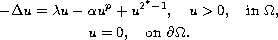where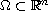,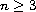is a bounded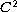-domain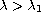,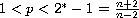and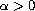is a bifurcation parameter. Brezis and Nirenberg  showed that a lower order (non-negative) perturbation can contribute to regain the compactness and whence yields existence of solutions. We study the equation with an indefinite perturbation and prove a bifurcation result of two solutions for this equation.

Submitted August 12, 2005. Published October 25, 2006.
Math Subject Classifications: 49K20, 35J65, 34B15.
Key Words: Critical Sobolev exponent; positive solutions; bifurcation.

Show me the PDF file (232K), TEX file, and other files for this article.Yuanji Cheng School of Technology and Society Malmo University SE-205 06 Malmo, Sweden email: yuanji.cheng@ts.mah.se